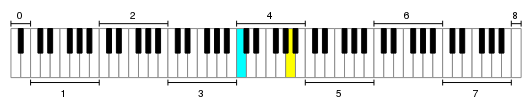# Blog » Renold-II Temperament (“The Scale of Fifths”) by Maria Renold

Reading Time: 4 minutes April 20, 2016

#### RENOLD-II TEMPERAMENT (“THE SCALE OF FIFTHS”)

(by Maria Renold, revised by Peter Stevens & Paul Davis)

In an earlier article on this blog I have written about and explained the “Renold-I” temperament, the first version of the “Scale of Fifths” that Maria Renold made in 1962. If you have not read that article yet, then I suggest you do so, just to be able to place everything in perspective.

After Maria Renold created – what later would be known as the “Renold-I” temperament – she continue experimenting and developing her concept. The result is what is known as the “Renold-II” (also known as “Middle Tuning” temperament.

##### WHAT IS DIFFERENT ABOUT RENOLD II?

The most important difference between “Renold-I” and “Renold-II” is the type of “Musical Interval System” (the range of notes within the system / the number of actual tones available to use). In the case of “Renold I” a closed system is used, the “Renold-II” uses an “open system“.

What does that mean?

##### CLOSED SYSTEM (RENOLD-I)

Well, generally with a “closed system” the octaves are perfect doublings: C4=256Hz thus C5=512Hz, a difference of 1200 cents. In this tuning system there are 12 tones per octave and every octave includes a perfect doubling of those 12 tones.Let’s look at the piano to visualize it. A standard acoustic piano has 88 keys. This covers 7 octaves with 12 tones (=84), +4 semitones.

From from every tone of the 12-tone scale there are 7 (or 8) exact doubles/halves. So, if we take for example the tone C4=256Hz as reference, then we have a perfect doubling at:

C1=32Hz, C2=64Hz, C3=128Hz, C4=256Hz, C5=512Hz, C6=1024HZ, C7=2048Hz and C8=4096Hz.

All these “C’s” are “identical”, they are the same frequencies doubled or halved. When you would play two or more perfect octaves (1200 cents precisely) of for example those “C’s” simultaneously, most people would no longer differentiate between the “C’s” but hear them as one.

Another reason why I call them “identical” is that they are all part of the same harmonic series. If we take for example C1 as the “fundamental” (the first harmonic), then C2 aligns perfectly with the 2nd harmonic of C1, as do all other perfect octaves of C1: C3=4th harmonic, C4=8th harmonic, C5=16th harmonic, C6=32nd harmonic, C7=64th harmonic and C8=128th harmonic. From C2 we could do exactly the same and those “C’s” above C2 would align in the same relative fasion to C2 as the “C’s” above C1 did to C1.

To visualize a closed system you could draw it as a circle.

##### OPEN SYSTEM (RENOLD-II)

In the case of this “open system” the octave is not a perfect doubling but slightly larger, (1203.35 cents to be precise). The consequence of this is that the 12 tones of the scale no longer “double”. If we set C4=256Hz as reference, then we have the following:

C1=31.815Hz, C2=63.753Hz, C3=127.753Hz, C4=256Hz, C5=512.992Hz, C6=1027.971Hz, C7=2059.923 and C8=4127.927Hz.

The consequence of this is that we end up having 88 “different” tones on the piano. To visualize an open system, you could draw it as a spiral.

##### RENOLD-II @ A4=432Hz

Below a part of the Renold-II temperament. To add the complete range (C0-C9) would be too much for this page. You can though download the full range of tones and frequencies in a PDF file.

A4=432Hz has been used as reference pitch for the example table in this article and the downloadable PDF file because this temperament is mostly used by members of the 432-community. If you wish to use another concert pitch you have to do “the math” yourself. This is all you need to know for it:

• In the 1st column you see “-1203.35 down” and “1203.35 up“. This is the amount of cents up or down from every tone from C4 to B4.

Example: A4=432Hz -1203.35 cents = 215.5824363807 Hz (A3)

• In the 2nd column you see a series of cent corrections for the tones C4 – B4  compared to Equal Temperament.
• The 3rd column containes the tone names.
• The frequencies in the 4th column are C4 to B4 in 432Hz 12-TET
• In column 5 you see the corrected tone frequencies (Renold-II).
 TONE CORRECTION TONE FREQUENCIES -1203.35 down 1203.35 up … … … G♯3 / A♭3 203.88 203.3163932923 A3 216 215.5824363807 A♯3 / B♭3 228.84 228.5873823048 B♭3 242.45 242.3715877002 -5.874 C4 256.87 255.99992842560002 -4.47 C♯4 / D♭4 272.14 271.4382481425206 -3.07 D4 288.33 287.819157041 -1.68 D♯4 / E♭4 305.47 305.1737138452 -0.28 E4 323.63 323.5776621474 -5.59 F4 342.88 341.7746566158 -4.19 F♯4 / G♭4 363.27 362.3918628923 -2.79 G4 384.87 384.2502556477 -1.4 G♯4 / A♭4 407.75 407.4203973981 0 A4 432 432 1.4 A♯4 / B♭4 457.69 458.0602706488 2.79 B♭4 484.9 485.6820789499 C5 513.74 512.9915541197 C♯5 / D♭5 544.29 543.9279988027 … … …

IMPORTANT NOTES

• The recommended concert pitch for this temperament is C3=128Hz (C4=256Hz & A4=432Hz). Reason of it is explained in Maria Renold’s book on page 69.
• The frequencies in the table on in this article are only a “guideline“. For proper results fine tuning should be done by ear (according to scheme included in Maria Renold’s book). For tuning by ear three tuning fork are required: C4=256HzA4=432Hz and “gelis” (F#/Gb)=362,40Hz.
• It might be good to mention that the Renold-II temperament doesn’t sound (as well as Renold-I) on synthesizers and other electronic devices! The Renold-II temperament does work well for acoustic instruments.